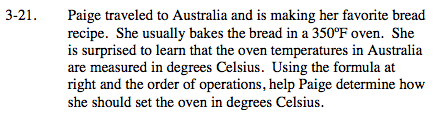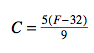### Home > MC2 > Chapter 3 > Lesson 3.1.2 > Problem3-21

3-21.We are given degrees Fahrenheit and we need degrees Celsius.

Substitute 350°F for the variable F.
Then solve within the parentheses.

Simplify the numerator by multiplying.$C = \frac{5(350 - 32)}{9}$
$C = \frac{5(318)}{9}$
$C = \frac{1590}{9}$